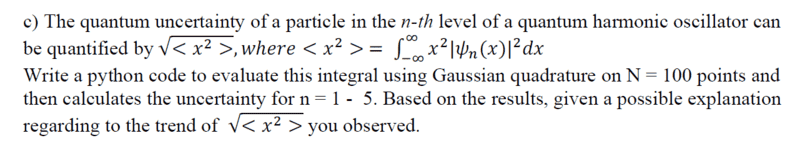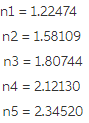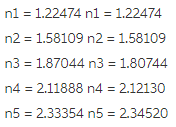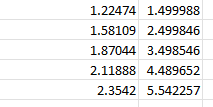# The Quantum Uncertainty of a Particle

maguss182
Thread moved from the technical forums to the schoolwork forums
Summary:: I'm trying to understand the meaning behind the answer of 2.34... but I haven't taken a quantum class yet so I'm utterly lost.

So I took this physics class that's teaching us python and for our final project our teacher assigned random problems to work out, well I got stuck with a quantum harmonic oscillator problem, and while I worked out the code easy enough, the final question I have no idea how to answer it.The answer I get is 2.34... but I'm not sure what the trend is? It gets closer and closer to 2.34? Why? Any help would be greatly appreciated, thanks!

Homework Helper
Gold Member
They are asking if you can see a trend in the numbers that you get for ##\sqrt{<x^2>}## for ##n = 1, 2, 3, 4, 5##.

This might be hard to recognize. However, try looking at the square of these numbers and see if you recognize a trend.

•BvU
maguss182
Well I see that as we get to 5 the answer gets closer to 2.34,Is it saying that as the number goes up the uncertainty gets closer and closer to 2.34? So a more precise calculation?

Homework Helper
Gold Member
What do the squares of these numbers look like?

maguss182Ok so they are almost the same thing right?

Homework Helper
Gold Member
Calculate (1.22474)2, (1.58109)2, etc.

maguss182Seems to increase by ~1? Sorry I'm so lost, math is not my strong suit

maguss182
wait uncertainty increases as n increases

Homework Helper
Gold Member
View attachment 293955

Seems to increase by ~1? Sorry I'm so lost, math is not my strong suit

Yes, the numbers in the second column increase by 1. You are doing numerical integration. So, you know it is not perfectly accurate and will tend to get less accurate as n increases (because ##\psi_n(x)## varies more rapidly as n increases). Suppose you round off your second column of numbers to the nearest tenth. Can you guess what the exact values of these numbers would be if there wasn't any error in the numerical integration?

Note that the second column of numbers represents ##<x^2>## for the states ##n = 1, 2, 3, 4, 5##.

Can you guess the exact formula for ##<x^2>## as a function of ##n##?

Homework Helper
Gold Member
wait uncertainty increases as n increases
Yes. Could this be the only trend that they wanted you to notice? I don't know. If so, then you can ignore what I was trying to get you to see.maguss182
I think so lol sorry, thinking on what you said though would it be the quantum harmonic oscillation? that is what the problem is about

Homework Helper
Gold Member
I think so lol sorry, thinking on what you said though would it be the quantum harmonic oscillation? that is what the problem is about
You mentioned that you have not taken a quantum mechanics course. So, you might not be familiar with the energy levels, ##E_n##, of the harmonic oscillator: $$E_n = hf (n+1/2) \,\,\,\,\,\,\, n = 1, 2, 3, 4, ...$$ where ##h## is Planck's constant and ##f## is the frequency of the oscillator.

For a specific oscillator, you can choose units such that ##hf = 1##. Then $$E_n = n+1/2$$
You can show that in these units ##<x^2>## for a particular quantum state ##\psi_n(x)## actually equals ##E_n##. So, ##\sqrt{<x^2>} = \sqrt{E_n} = \sqrt{n+1/2}##.

Hope I haven't caused too much confusion. As I said, you can ignore all of this if it's something you are not familiar with.

maguss182
Oh ok yeah no I haven't taken a course yet so that's new to me but thanks for all your help, I was able to figure out my question, thank you!

Homework Helper
Gold Member
Oh ok yeah no I haven't taken a course yet so that's new to me but thanks for all your help, I was able to figure out my question, thank you!
OK, great.